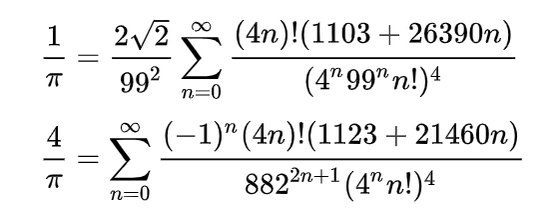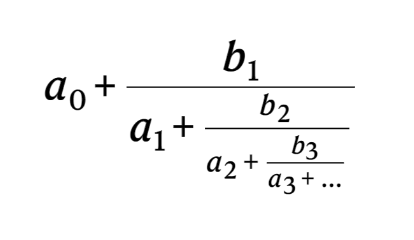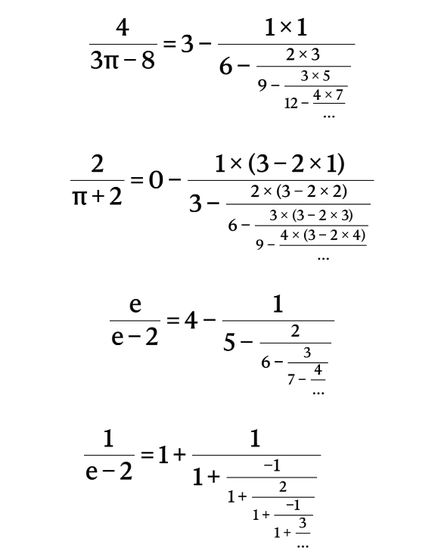# The AI 'Ramanujan Machine' that predicts and creates mathematical formulas like the genius mathematician Ramanujan is bornIsrael Institute of Technology, AI ' Ramanujan Machine ' that finds mathematical formulas like the genius mathematician

Srinivasa Ramanujan who got the nickname of 'Indian magician' by discovering many mathematical formulas by genius inspiration Developed by a university research team.

Generating conjectures on fundamental constants with the Ramanujan Machine | Nature
https://www.nature.com/articles/s41586-021-03229-4

AI maths whiz creates tough new problems for humans to solve
https://www.nature.com/articles/d41586-021-00304-8

World's hottest new math mind-teasers set by Israeli AI formula-finder | The Times of Israel
https://www.timesofisrael.com/worlds-hottest-new-math-mind-teasers-set-by-israeli-ai-formula-finder/

Machines Are Inventing New Math We've Never Seen
https://www.vice.com/en/article/xgzkek/machines-are-inventing-new-math-weve-never-seen

Ramanujan discovered nearly 4000 theorems and mathematical formulas such as the following pi formula, and answered the reason why it was too intuitive, such as 'The goddess taught me while sleeping' about the proof. It is also known as the 'Indian Wizard'. Ramanujan died early in 1920 at the young age of 32, but the verification of all theorems and mathematical formulas he discovered took up to 80 years after his death, and many of them were correct, so he was 'a genius who illuminated the future of mathematics.' Was also said.The 'Ramanujan Machine', named after Ramanujan, is an AI that predicts

irrational numbers such as pi and Napier's number e in the form of a denominator called a continued fraction as shown below.The continued fraction predicted by Ramanujan Machine is characterized by having a certain regularity in the above a 0・ a 1・ a 2 and b 0・ b 1・ b 2 parts. The equations actually predicted are as follows, and the numerical values corresponding to a n and b n have regularities such as “1,2,3,4 ……” and “3,6,9,12 ……”. At this point, the task of proving the predicted mathematical formulas is left to human mathematicians.Ramanujan Machine has already predicted 19 formulas, and the formulas for Catalan numbers have proved to be more accurate than those found so far, but the formulas for Apery's constant have clues to prove. It has not been found. Id Kaminer, who leads the project, believes that if he can develop a smartphone app version of Ramanujan Machine and utilize the arithmetic processing of smartphones that exist all over the world, he will be able to discover more mathematical formulas.

in Software, Posted by log1k_iy